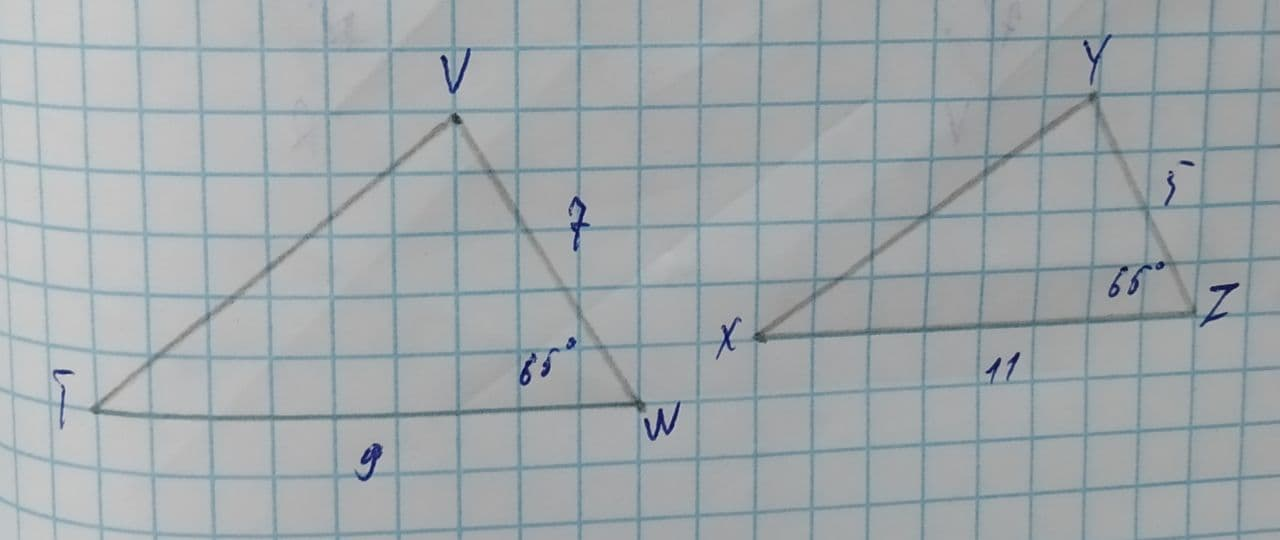# Determine (if possible) the similarity postulate that proves the following two triangles are similar:a2linetagadaW 2021-07-30 Answered
Determine (if possible) the similarity postulate that proves the following two triangles are similar:SAS similarity postulate
AA similarity postulate
SSS similarity postulate
These triangles are not similar
You can still ask an expert for help

• Questions are typically answered in as fast as 30 minutes

Solve your problem for the price of one coffee

• Math expert for every subject
• Pay only if we can solve itClara Reese
In the given triangles $\mathrm{△}TVW$ & $\mathrm{△}XYZ$.
$m\mathrm{\angle }VWT\stackrel{\sim }{=}m\mathrm{\angle }YZX\stackrel{\sim }{=}{65}^{\circ }$
The ratio of corresponding sides of triangles.
$\frac{\stackrel{―}{VW}}{\stackrel{―}{YZ}}=\frac{7}{5}$
$\frac{\stackrel{―}{TW}}{\stackrel{―}{XZ}}=\frac{9}{11}$
$\because \frac{\stackrel{―}{VW}}{\stackrel{―}{YZ}}\ne q\frac{\stackrel{―}{TW}}{\stackrel{―}{XZ}}$
$\because$ ratio of corresponding sides are not equal, hence triangles are not similar.